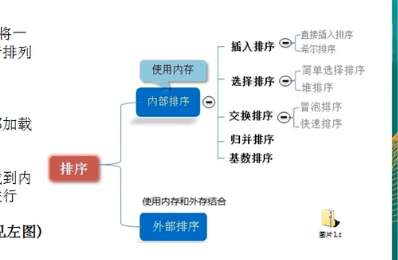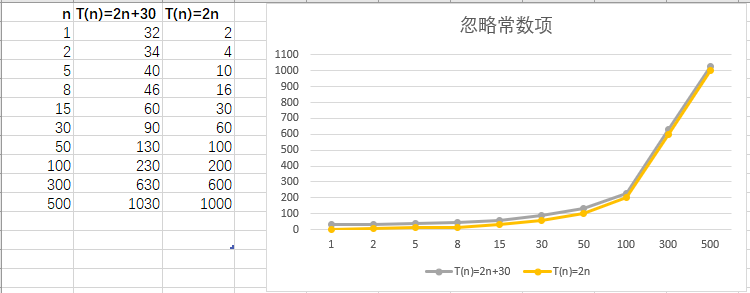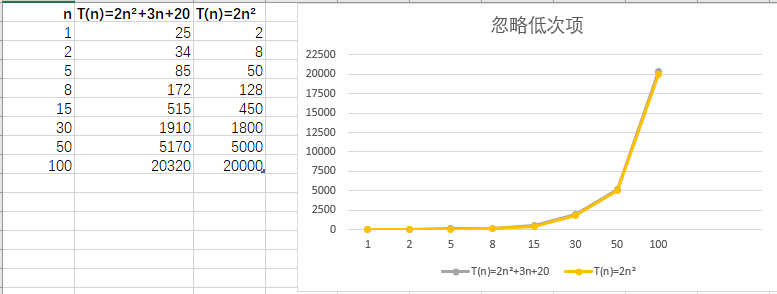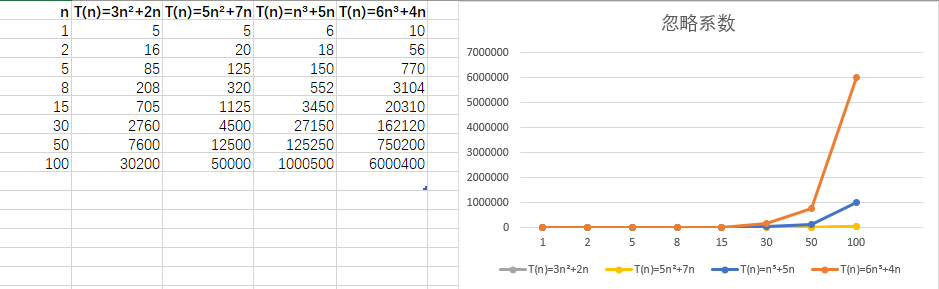• **时间频度：**一个算法花费的时间与算法中语句执行次数成正比。 对于时间频度来说，常数项可以忽略，低次项也可以忽略， 时间复杂度：**时间频度：**一个算法花费的时间与算法中语句执行次数成正比。 对于时间频度来说，常数项可以忽略，低次项也可以忽略，
展开全文• 一个算法执行所耗费的时间，从理论上是不能算出来的，必须上机运行测试才能知道。...一个算法中的语句执行次数称为语句频度或时间频度。记为T(n)。算法的时间复杂度是指执行算法所需要的计算工作量。 ...
一个算法执行所耗费的时间，从理论上是不能算出来的，必须上机运行测试才能知道。但我们不可能也没有必要对每个算法都上机测试，只需知道哪个算法花费的时间多，哪个算法花费的时间少就可以了。并且一个算法花费的时间与算法中语句的执行次数成正比例，哪个算法中语句执行次数多，它花费时间就多。一个算法中的语句执行次数称为语句频度或时间频度。记为T(n)。算法的时间复杂度是指执行算法所需要的计算工作量。
展开全文• 时间频度与时间复杂度的概念及计算


文章目录
一、时间频度1. 概念2. 特点2.1 忽略常数项2.2 忽略低次项2.3 忽略系数

二、时间复杂度1. 概念2. 计算时间复杂度3. 常见的时间复杂度3.1 常数阶 O(1)3.2 对数阶 O(㏒n)3.3 线性阶 O(n)3.4 线性对数阶 O(n㏒n)3.5 平方阶 O(n^2)
4. 平均时间复杂度与最坏时间复杂度

一、时间频度
1. 概念

一个算法花费的时间与算法中语句的执行次数成正比例，哪个算法中语句执行次数多，它花费的时间就多。一个一个算法中的语句执行次数称为语句频度或时间频度，记为 T(n)。

如：
计算 1~100 所有数字之和，有以下两种方式：
第一种方式：
int total = 0;
for (int i = 1; i <= 100; i++) {
total += i;
}
其时间频度 T(n) = n+1 （当 i = 101 时，for 循环还会进行一次判断，所以为 n+1）

第二种方式：
int total = 0;
total = (1 + 100) * 100 / 2;
其时间频度 T(n) = 1

2. 特点

随着程序规模的增大，时间频度有以下三个特点：
忽略常数项忽略低次项忽略系数 （n^k (k>=3)不适用）

2.1 忽略常数项

由下图可知： 随着程序规模 n 的增大，T(n) = 2n+30 与 T(n) = 2n 的执行曲线无线接近，常数项 30 可以忽略不计。2.2 忽略低次项

由下图可知： 随着程序规模 n 的增大，T(n) = 2n²+3n+20 与 T(n) = 2n² 的执行曲线基本重合，低次项 3n+20 可以忽略不计。2.3 忽略系数

由下图可知： 随着程序规模 n 的增大，T(n) = 3n²+2n 与 T(n) = 5n²+7n 的执行曲线基本重合，系数 3 和 5 可以忽略不计。 但是 T(n) = n³+5n 与 T(n) = 6n³+4n 执行曲线分离，所以忽略系数不适用于 n^k(k>=3) 的情况。二、时间复杂度
1. 概念

一般情况下，算法中的基本操作语句的重复执行次数是问题规模n的某个函数，用 T(n) 表示，若有某个辅助函数 f(n) ，使得当 n 趋近于无穷大时，T(n) / f(n) 的极限值为不等于 0 的常数，则称 f(n) 是 T(n) 的同数量级函数，记作 T(n) = O(f(n)) ，称 O(f(n)) 为算法的渐进时间复杂度，简称时间复杂度。

2. 计算时间复杂度

T(n) 不同，时间复杂度可能相同。

如：
T(n) = n^2+7n+6 与 T(n) = 3n^2+2n+3 的 T(n) 不同，
但是它们的时间复杂度相同，都为 O(n^2)，
忽略常数项，忽略低次项、忽略系数，两者时间频度都为 T(n) = n^2，
存在辅助函数 f(n) = n^2 使得 T(n) / f(n) = 1 （不为0的常数）
所以，T(n) = n^2+7n+6 与 T(n) = 3n^2+2n+3 的时间复杂度都为 O(f(n)) = O(n^2)

3. 常见的时间复杂度

常见的算法时间复杂度由小到大依次为：O(1)<O(㏒n)<O(n)<O(n㏒n)<O(n^ 2)<O(n^ 3) <O(n^ k)<O(2^ n)，随着问题规模 n 的不断增大，上述时间复杂度不断增大，算法的执行效率降低。

3.1 常数阶 O(1)

无论算法执行了多少行代码，只要是没有循环等复杂结构，那么该算法的时间复杂度都是 O(1).

如：
int i = 10;
int j = 20;
i++;
++j;
int s = i + j;
其时间复杂度为 O(1)

3.2 对数阶 O(㏒n)

如下所示，在 while 循环中，每次循环都会将 i 乘以 2，当循环 ㏒₂n 次之后，这段代码结束，所以其时间复杂度为 O(㏒₂n)。 对数阶的底数是基于代码变化的，假设循环中执行的代码为 i = i * 3 则这段代码的时间复杂度为 O(㏒₃n)。

如：
int n = 100;
int i = 1;
while (i < n) {
i = i * 2;
}
其时间复杂度为 O(㏒₂n)

3.3 线性阶 O(n)

如下所示，for循环中的代码会执行n次，这段代码所消耗的时间随着 n 的变化而变化，所以其时间复杂度为 O(n)。

如：
int n = 100;
int j = 1;
for (int i = 0; i < n; i++) {
j++;
}
其时间复杂度为 O(n)

3.4 线性对数阶 O(n㏒n)

如下所示，内层循环的时间复杂度为 O(㏒₂n)，外层循环的时间复杂度为 O(n)，所以这段代码的时间复杂度为 O(㏒₂n) * O(n)，即 O(n㏒₂n)。

如：
int n = 100;
for (int i = 0; i < n; i++) {
int j = 1;
while (j < n) {
j = j * 2;
}
}
其时间复杂度为O(n㏒₂n)

3.5 平方阶 O(n^2)

嵌套两层时间复杂度为 O(n) 的循环，其时间复杂度就为 O(n^2)。 立方阶和 k 次方阶与之类似。

如：
int n = 100;
int s = 0;
for (int i = 0; i < n; i++) {
for (int j = 0; j < n; j++) {
s++;
}
}
其时间复杂度为 O(n^2)

4. 平均时间复杂度与最坏时间复杂度

平均时间复杂度是指所有可能的输入实例均以等概率出现的情况下该算法的运行时间。 最坏时间复杂度是指最坏情况下该算法的运行时间。 一般讨论的时间复杂度都是最坏情况下的时间复杂度，因为最坏情况下的时间复杂度是算法在任何输入实例上运行时间的界限，这就保证了算法的运行时间不会比最坏情况更长。

常用排序算法的时间复杂度：
排序法平均时间复杂度最坏时间复杂度冒泡排序O(n²)O(n²)交换排序O(n²)O(n²)选择排序O(n²)O(n²)插入排序O(n²)O(n²)希尔排序O(n㏒n)O(n^s) 1<s<2快速排序O(n㏒n)O(n²)归并排序O(n㏒n)O(n㏒n)堆排序O(n㏒n)O(n㏒n)
展开全文算法 数据结构 java
• 度量一个程序算法执行时间的两种方法 事后统计法: 直白就是把程序跑一边，统计程序从开始到结束花费的时间。...一个算法中语句执行的次数成为时间频度，记做T(n)。 例如：计算1-100的总和 // 方式1 int t
度量一个程序算法执行时间的两种方法
事后统计法: 直白就是把程序跑一边，统计程序从开始到结束花费的时间。缺点在于需要将程序跑一边，如果越到耗时程序的时候效率不高，而且要求计算机的硬件软件的环境一致，保证运行环境相同才有可信度。事后统计法: 通过分析某一个程序算法具体的时间复杂度来度量一个算法的优劣。
时间频度
一个算法花费的时间与算法中语句执行数成正比。哪个算法执行的次数越多，则它花费的时间就多。一个算法中语句执行的次数成为时间频度，记做T(n)。
例如：计算1-100的总和
// 方式1
int total = 0;
int num = 100;
for(int i=0;i<=num;i++){
total = i+total;
}

// 方式2
int num = 100;
int total = (1+num)*num/2

此时方式1中的时间频度为100+1次 记为 T(n)=n+1 n=100因为循环了100次 +1是因为有一个判断
方式2的时间频度为1 因为只有一条语句 记为 T(n)=1
时间复杂度
时间复杂度是度量算法花费时间，算法中的基本操作语句和重复执行次数是问题规模N的某个函数，用T(n)表示，若有有个辅助函数f(n)，当n趋近无穷大时，T(n)/f(n)的极限值为一个不等于零的常数，则称f(n)和T(n)是同数量级的函数记为 T(n)=O(f(n))，简称O(n)，为一个算法的渐进时间复杂度，简称时间复杂度。
时间复杂的计算方法
分析出算法的运行时间频度用常数1代替算法中所有加法常数 如 T(n)=2n²+5n+5 ==> T(n)=2²+5n+1只保留最高阶项 如 T(n)=2n²+5n+5 ==> T(n)=2n²取出最高阶的系数 T(n)=2n²+5n+5 ==> T(n)=n² 则最终时间复杂度为 T(n)=n²记为O(n²)
常见时间复杂度
[外链图片转存失败,源站可能有防盗链机制,建议将图片保存下来直接上传(img-8OhRClVG-1594869812967)(C:\Users\denglw\AppData\Roaming\Typora\typora-user-images\image-20200715103543819.png)]
常数阶 O(1) 无论代码执行了多少行，只要没有循环等复杂结构时间复杂度为O(1) int i = 1;
int j = 2;
i++;
j++;
int total = i+j;
 ​ 上述代码时间消耗不会随着变量的增长而增长，即i=1或者i=10000都是一样的，无论有多少行，一行或者及万行都可以用O(1)来表示时间复杂度。  对数阶 O(log2n) int i = 1;
int n = 1024;
while(i < n){
i = i*2;
}
// 或者
for(int i=0; i<n; i*=2){
System.out.println(i);
}
 上述代码中while循环或者for 循环 的执行次数为 以2为底数1024的对数 log2N，当N增大时，时间频度成对数上升，类似于一根长16M的绳子，每次割一半，割到一米需要几次的问题，第一次为8M，第二次为4M，第三次为2M，第四次为1M，则时间频度具体值为4,为log16。则可以记做O(logn)。上述如果把i=i2改成 i=i3 则变成了 log3N 以3为底N的对数了.  线性阶 O(n) int n =100;
for(int i=0; i<n; i++){
System.out.println(i);
}
 则表示for循环中需要执行多少次，上述代码是一个线性的，随着N的增加，执行次数就增加N次。如n=100 则执行100次 n=200则执行200次，在坐标轴上是一条直线上升的趋势。记为O(n)  线性对数阶 O(nlog2n) int n =100;
int m = 1024;
for(int i=0; i<n; i++){
while(i < m){
i = i*2;
}
}
 简单的理解就是一个对数阶的代码循环N遍则变成了线性对数阶，上述代码中 while循环是一个对数阶 为log1024 记做logN for循环是一个线性阶 n n=100 则他们一起的时间复杂度为O(nlogN)  平方阶 O(n^2) int n = 100;
int m = 100;
for (i=0; i < n ; i++){
for(i=0; i < m ;i++){
System.out.println(i);
}
}
 平方阶就是循环套循环，2层循环就是一个n2的平方阶，时间复杂度就是O(n2)  立方阶 O(n^3) 立方阶则是3层循环嵌套  k 次方阶 O(n^k) k层循环嵌套  指数阶 O(2^n) long function1(int n) {
if (n <= 1) {
return 1;
} else {
return function1(n - 1) + function1(n - 2);
}
}
 类似上述从出现递归的时候，T(0) = T(1) = 1，同时 T(n) = T(n - 1) + T(n - 2) + 1，这里的 1 是其中的加法算一次执行。显然 T(n) = T(n - 1) + T(n - 2) 是一个斐波那契数列，通过归纳证明法可以证明，当 n >= 1 时 T(n) < (5/3)^n，同时当 n > 4 时 T(n) >= (3/2)^n。所以该方法的时间复杂度可以表示为 O((5/3)^n)，简化后为 O(2^n)。
上述时间复杂度从上到下一次递增，在程序设计过程中需要避免指数阶的时间复杂度。
空间复杂度
指程序算法运行过程中对临时占用存储空间如内存的大小，不过从用户体验来是说，用户并不关系后台占用的具体多少内存，只关心多久能给我反馈即执行的速度，所以出现了很多缓存产品，本质上就是用空间换时间的过程。
展开全文• Temporal Frequency& Time Complexity& Space Complexity计算算法执行时间时间复杂度(Time Complexity)常数阶O...时间频度(Temporal Frequency): 一个算法所花费的时间与算法中语句的执行次数成正比. 算法中的算法时间复杂度 算法空间复杂度
• 时间频度 一个算法花费的时间与算法中语句的执行次数成正比，哪个算法中语句执行次数多，它花费时间就多。一个算法中的语句执行次数称为语句频度或时间频度。记为T(n)。 时间复杂度 一般情况下，算法中的基本操作...时间复杂度 算法
• 时间频度：T(n) 一个算法中的语句执行次数称为语句频度或时间频度。记为T(n)。 时间复杂度：O(f(n)) T(n)=O(f(n)),称O(f(n)) 为算法的渐进时间复杂度，简称时间复杂度。
• 时间频度是指算法中最基本的语句执行的次数叫做时间频度； 2.什么是时间复杂度？ 时间复杂度是指当需要处理数据的规模发生变化的时候，计算机计算次数的变化趋势叫做时间复杂度；（而且，我么你需要知道时间...算法 算法导论
• （1）时间频度一个算法执行所耗费的时间，从理论上是不能算出来的，必须上机运行测试才能知道。但我们不可能也没有必要对每个算法都上机测试，只需知道哪个算法花费的时间多，哪个算法花费的时间少就可以了。并且一...
• 排序算法分类和介绍、时间频度、时间复杂度1. 排序算法分类和介绍2. 算法的时间复杂度3. 算法的空间复杂度 1. 排序算法分类和介绍 排序算法的介绍 排序也称排序算法(Sort Algorithm)，排序是将一组数据，依指定的...
• 时间频度：一个算法花费的时间与算法中语句的执行次数成正比例，哪个算法中语句执行次数多，它花费时间就多。一个算法中的语句执行次数称为语句频度或时间频度。记为T(n) 时间复杂度 1)一般情况下，算法中的基本...数据结构
• 一、时间频度 定义：一个算法花费的时间与算法中语句的执行次数成正比例，哪个算法中语句执行次数多，它花费时间就多。一个算法中语句的执行次数称为语句频度或时间频度，记为T(n). 实例：计算1~100的和。 注：第一...
• <!-- TOC --> <li><a href="#1-%E6%97%B6%E9%97%B4%E9%A2%91%E5%BA%A6">1. 时间频度</a><ul> <li><a href
• 一、时间频度基本介绍 时间频度：一个算法花费的时间与算法中语句的执行次数成正比例，哪个算法中语句执行次数多，它花费时间就多。一个算法中的语句执行次数称为语句频度或时间频度。记为T(n)。 二、时间频度特点...
• s=0; for(i=1;i;i++) for(j=0;j;j++) s+=j; 求出语句执行频度c语言
•算法 数据结构 java
• ## 计算语句频度

千次阅读 多人点赞 2020-08-06 23:16:01
时间频度 一个算法执行所耗费的时间，从理论上是不能算出来的，必须上机运行测试才能知道。但我们不可能也没有必要对每个算法都上机测试，只需知道哪个算法花费的时间多，哪个算法花费的时间少就可以了。并且一个...c语言 数据结构
•文档
• 源代码如下，这个时间复杂度为什么不是O(n)而是O(Ln(n))？ 此外，这道题的频度T(n)又是多少？  while(n!=0) { n=n/2; }源代码 算法
• 要计算 算法的时间复杂度 ，先要掌握什么是算法的频度 算法频度就是基本操作执行次数的总和 （f(n) n是执行问题的规模 ） 再对整个算法的频次进行以下操作： 去掉常数项 只保留最高阶项 这就是时间复杂度 T(n) ，...数据结构
• 2.3. a=0; b=1; ① for (i=1;i;i++) ② { s=a+b;　③ b=a;　④ a=s;　⑤ } 我觉得是：1. 频度=2 2.频度=n+1 3.4.5. 频度=n 没毛病吧？数据结构与算法
• 语句频度 T(n)称为时间频度，指的是该语句重复执行的次数 例题一： 矩阵相乘的关键代码 1 for(int i=0;i<n;i++) n+1 2 for(int j=0;j<n;j++) n(n+1) 3 c[i][j]=0; n*n 4 for(int k=0;...
• 某型公共汽车主要系统的维修活动类型频度修理时间的统计分析毕业论文.doc文档
• 其中的f(n)一般是算法中频度最大的语句频度。 主要用算法时间复杂度的数量级(即算法的渐近时间复杂度)评价一个算法的时间性能。 常见的时间复杂度按数量级递增排列依次为：常数0(1)、对数阶0(log 2 n)、线形阶0(n)...
• 平均时间复杂度为 O(n)； 代码 # include # include # include # include using namespace std; int main() { int a[] = {1,3,2,3,4,3,6,3,3}; int len = sizeof(a) / sizeof(int); nth_element(a,...
• （1）时间频度 一个算法执行所耗费的时间，从理论上是不能算出来的，必须上机运行测试才能知道。但我们不可能也没有必要对每个算法都上机测试，只需知道哪个算法花费的时间多，哪个算法花费的时间少就可以了。并且一...算法 空间复杂度...